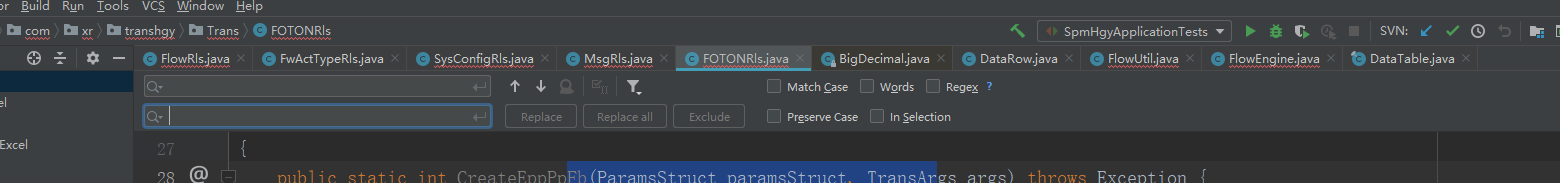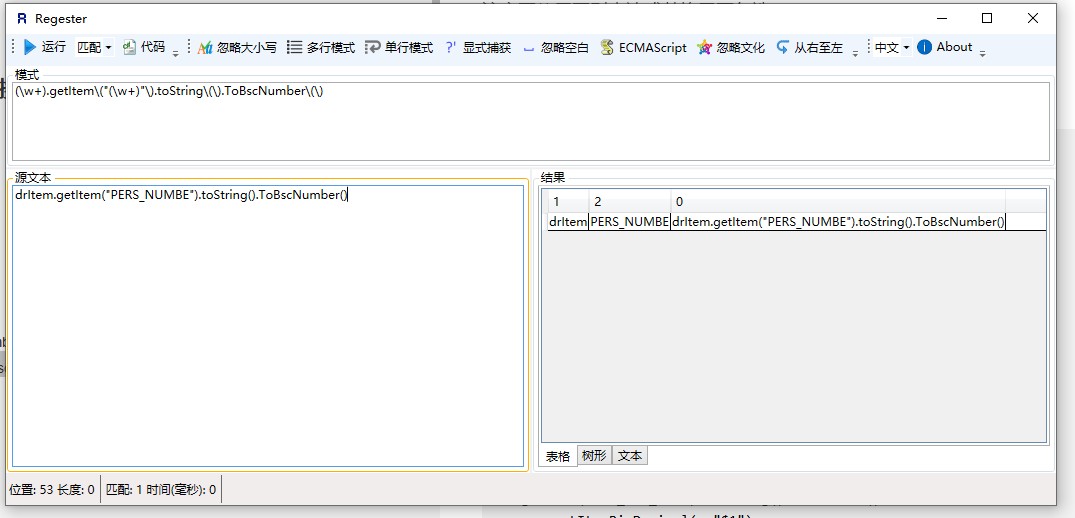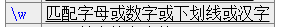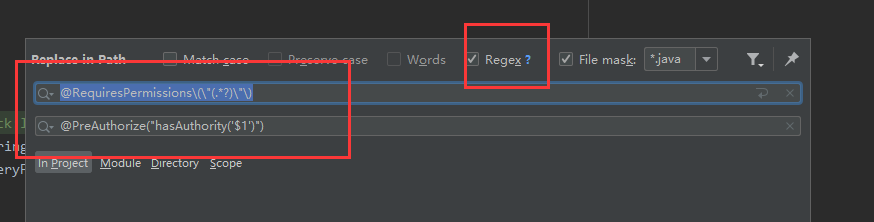• ## idea正则表达式替换

千次阅读 2019-05-16 20:56:48
'm','orderNum','s') ，这个函数的调用有很多地方，有一天我们想给这个函数加一个默认参数，让它变成 getView(o,'m','orderNum','s','*')，一个一个加太麻烦了，我们就用 idea 自带的正则表达式替换功能来实现。...
例如，现在有一个函数 getView(o,'m','orderNum','s') ，这个函数的调用有很多地方，有一天我们想给这个函数加一个默认参数，让它变成 getView(o,'m','orderNum','s','*')，一个一个加太麻烦了，我们就用 idea 自带的正则表达式替换功能来实现。

首先按 Ctrl + R ，打开替换对话框，然后在上面输入 getView$$(.+)$$，下面输入 getView($1,'*')，然后就能全部替换了。 这里用了正则表达式的组匹配，getView$$(.+)$$ 红色部分我们匹配了一个组，内容是 getView(o,'m','orderNum','s')。getView($1,'*') 这个 红色部分 $1 代表匹配的第一个内容，这样就实现了正则替换。  展开全文• 注意要使用正则表达式替换需要勾选regex 2.利用正则表达式进行替换 下面展示一些 简单操作的例子。 (\w+)get$$0$$.getValue$$"(\w+)"$$ dtCfwStget(0).getValue("CFW_CD")$1.getValue(0,"$2") dtCfwSt.... idea 正则表达式搜索替换简单应用 利用正则表达式，idea可以进行快速大量替换 1.CTRL+R 快捷键调出下面当前页面替代操作栏注意要使用正则表达式替换需要勾选regex 2.利用正则表达式进行替换 下面展示一些 简单操作的例子。  (\w+)get$$0$$.getValue$$"(\w+)"$$ dtCfwStget(0).getValue("CFW_CD")$1.getValue(0,"$2") dtCfwSt.getValue(0,"CFW_CD") (\w+).getItem$$"(\w+)"$$.toString.ToBscNumber drItem.getItem("PERS_NUMBE").toString().ToBscNumber()$1.getItemBscNumber("$2") drItem.getItemBscNumber("PERS_NUMBE") : (\w+).Rows\) for (DataRow drItem : dtCardInfo.Rows) :$1.Rows())
for (DataRow drItem : dtCardInfo.Rows())

row.getItem$$"(\w+)"$$.toString.ToDecimal
row.getItem("ACT_MTH_VAL").toString().ToDecimal()
row.getItemBigDecimal(	"$1") row.getItemBigDecimal( "ACT_MTH_VAL") $0$$"(\w+)"$ matAssResRows["ASS_VAL"] .get(0).getItem("$1")
matAssResRows.get(0).getItem("ASS_VAL")

以第二个为例（regester很好用）若果想把类似于
drItem.getItem("PERS_NUMBE").toString().ToBscNumber()
drItaaa.getItem("PERS_bbb").toString().ToBscNumber()
drItemcccc.getItem("PERS_NUMBEccccc").toString().ToBscNumber()

一件替换为
drItem.getItemBscNumber("PERS_NUMBE")
drItaaa.getItemBscNumber("PERS_bbb")
drItemcccc.getItemBscNumber(PERS_NUMBEccccc")


第一步 选取一条原代码，将所有的左括号，右括号，左中括号，右中括号前面叫上\ ，使其变为正确的正则表达式：
// drItem.getItem("PERS_NUMBE").toString().ToBscNumber();
// drItem.getItem$$"PERS_NUMBE"$$.toString.ToBscNumber;
具体还有哪些符号前面需要加\转义，可以网查

第二部 选择需要变为X 的变量，用（）选取，\w+指定选取括号里的字符根据需求选择，这里通用的为（/w+）代替 X
// X.getItem("X").toString().ToBscNumber();

// （/w+）.getItem$$"(\w+)"$$.toString.ToBscNumber;

这里匹配阶段结束（/w+）.getItem("(\w+)").toString().ToBscNumber()可以匹配到所有 X.getItem(“X”).toString().ToBscNumber()的代码
第三步替代  将你需要替代成的X表达式用$1,$2…替代
这是我最终需要代码的一种
//drItem.getItemBscNumber("PERS_NUMBE")

化为X表达式
// X.getItemBscNumber("X")

利用$加序号指定需要替代的位置 这是之前匹配阶段的X表达式，$1选中第一个（\w+）,，$2选中第一个（\w+）...... X.getItem("X").toString().ToBscNumber(); 将 X.getItemBscNumber("X")替代为$1.getItemBscNumber("$2")  最后输入替代这里报红是因为我替代过了  展开全文• 2.使用正则表达式 打开正则表达式功能 输入替换前的表达式和替换后的表达式 替换前 (.*) (.*)$1 代表第一个参数，这里代表第一个（.*) 就是id ,和name;$2同理 这里的 \n 有换行的作用 替换后 /**$2 */ \n...
要求
我们么有这样一堆数据
id 学生的学号
name 学生的名称

将数据转化为下面的格式粘贴到类中使用


步骤
1.alt + F （打开高级搜索）2.使用正则表达式

打开正则表达式功能输入替换前的表达式和替换后的表达式
替换前

(.*) (.*)


$1 代表第一个参数，这里代表第一个（.*) 就是id ,和name;$2同理
这里的 \n 有换行的作用

替换后
/** $2 */ \nprivate String$1 ;

3.替换4.效果
/** 学生的学号 */
private String id ;
/** 学生名称 */
private String name ;


拓展：默认贪心匹配，加上 ?（问号）就是非贪心匹配.


展开全文java
• idea 将 @RequiresPermissions("sys:ruletriggerlog:list") 替换为@PreAuthorize("hasAuthority('sys:ruletriggerlog:list')") @RequiresPermissions$$\"(.*?)\"$$ @PreAuthorize("hasAuthority('$1')") 全局... idea 将 @RequiresPermissions("sys:ruletriggerlog:list") 替换为 @PreAuthorize("hasAuthority('sys:ruletriggerlog:list')")@RequiresPermissions$$\"(.*?)\"$$ @PreAuthorize("hasAuthority('$1')")

全局替换即可

展开全文• UUID的标准形式 8-4-4-4-12 共32个字母或数字852f4dde-4dfa-4874-a9cb-a866eb4a5052不带杠的形式 共32个字符852f4dde4dfa4874a9cba866eb4a5052所使用的正则表达式 [a-z0-9]{8}[a-z0-9]{4}[a-z0-9]{4}[a-z0-9]{...
• 需要替换${aaa} 或${aaa.bbb} 使用方式： -------------------------------------------------- \$\{(([a-z]*\.[a-z]*)|([a-z]*))\} #($1) --------------------------------------------------
• 平常日常开发中，返回给前端的vo对象在字段上需要加注释@ApiModelProperty(“xxx”)，方便在前端同事在swagger...如果字段很多的话，一个一个写会比较耗时也枯燥，可以用正则表达式一键替。 /** * xxx */ （1）doma
• 在JetBrains IntelliJ IDEA,PHPStorm,WebStorm,Pycharm,CLion等中 用正则表达式 替换查找内容
• Ctrl+R全局搜索 正则表达式：public String getFileName\s\{[^}]*\} （public String ...替换正则表达式：public String getFileName() {\n\t\tString fileName = super.getFileName();\n\t\treturn...
• idea使用正则表达式查找并替换场景描述解决方法 idea构建maven工程，常常会修改pom文件，有时候要在多个位置更改相同字符串，特别是取消多个位置的注释，要一个个查找然后取消注释，比较麻烦。这里向大家推荐一个小...maven
• <p>The idea is to modify the query with this extra: <pre><code>AND m.id_module != 122 </code></pre> <p>So it looks like this: <pre><code> <p n="KeyFields"><![CDATA[]]></p><p n=...php regex
• 关于正则表达式 可以参考相应的笔记另外 如果要提取正则表达式中匹配到的内容，使用$1 -$... 按顺序取（第一个表达式 到 第N个表达式匹配到的数据）， 这点和linux正则获取的方式是一样的...
• 如下,我从doc文档把数据库表名复制到idea中java文件中,然后需要将变量名从下划线改成驼峰,又不能影响上面的注解内容. //原样 @Columu(name="i_aqabl_eqekn_cpdd") private String i_aqabl_eqekn_cpdd; @Columu(name...
• The overall idea is: <pre><code>$new = "stuff";$original = /*see below*/; $final = preg_replace('regex expression',$new, $original); </code></pre> <p>example of$original string: (...)"dl cm = ...php regex
• 原文匹配中文 excelMap.get$$(\"[\u4E00-\u9F15]+\")$$ 目标 excelMap.get$$1.hashCode\($$\) 转载于:https://www.cnblogs.com/cocoat/p/5850370.html
• 当您要搜索和替换特定的文本模式时，请...这里主要将一下，使用正则表达式进行文本的修改和替换替换时我们需要使用到捕获组，使用()表示，然后在IDEA中可以获取$n拿到捕获组中的值。 如: (\w+)-(\w+) 可以使用..字符串 • I had the idea to regex replace everything which is between (</code> and )</code> including the ()</code>: <pre><code>SELECT * FROM pages WHERE REPLACE(REGEXP('/$$[^\*].*$$/U'), '', page_title) = '...mysql php regex replace sql • ## Idea利用正则表达式 千次阅读 2019-12-17 11:55:55 正则表达式: . \w \s \d \b . 匹配除换行符以外的任意字符 \w 匹配字母或数字或下划线或汉字 等价于 '[^A-Za-z0-9_]'。 \s 匹配任意的空白符 \d 匹配数字 \b 匹配单词的开始或结束 ^ 匹配字符串的开始$ 匹配字符串...
• ... <pre><code>\$cfg['database'] = 'my_...<p>Can someone give me an idea of how to go about this, or point me to a good resource that would address this specific type of replacement situation? </div>php regex
• 背景说明 在项目中需要找到不规范代码进行全局查找，替换成规范代码。 例如：找到所有属性无注释的代码，idea中快捷键(ctrl+r) ...以上正则表达式详细说明： (?<!你的正则表达式) -》 标识不匹配的意思 \n ...
• 快捷键Ctrl+r 填入^\s*\n，勾选Regex，Replace all 转载于:https://www.cnblogs.com/DirWang/p/11263849.html